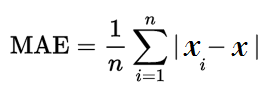# Mean Error: Definition

Share on

Statistics Definitions > Mean Error

## What is Mean Error?

The mean error is an informal term that usually refers to the average of all the errors in a set. An “error” in this context is an uncertainty in a measurement, or the difference between the measured value and true/correct value. The more formal term for error is measurement error, also called observational error.

## Why It’s Seldom Used

The mean error usually results in a number that isn’t helpful because positives and negatives cancel each other out. For example, two errors of +100 and -100 would give a mean error of zero:

mean = sum of all values/number in the set

= (+100 + -100) / 2

= 0 / 2 = 0.

Zero implies that there is no error, when that’s clearly not the case for this example.

To remedy this, use the mean absolute error (MAE) instead. The MAE uses absolute values of errors in the calculations, resulting in average errors that make more sense.The mean absolute error is usually used in experiments instead of the informal “mean error.”

The formula looks a little ugly, but all it’s asking you do do is:

1. Subtract each measurement from another.
2. Find the absolute value of each difference from Step 1.
3. Add up all of the values from Step 2.
4. Divide Step 3 by the number of measurements.

For a step by step example, see: mean absolute error.

## Other Terms that are Very Similar

While the “mean error” in statistics usually refers to the MAE, it could also refer to these closely related terms:

CITE THIS AS:
Stephanie Glen. "Mean Error: Definition" From StatisticsHowTo.com: Elementary Statistics for the rest of us! https://www.statisticshowto.com/mean-error/
---------------------------------------------------------------------------Need help with a homework or test question? With Chegg Study, you can get step-by-step solutions to your questions from an expert in the field. Your first 30 minutes with a Chegg tutor is free!